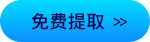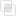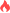python如何对文本进行拆分和写入

Python2021-04-22 17:23:52936浏览 · 0收藏 · 0评论1、拆分和写入过程

2、实例

```open_diff = open('data.txt', 'r') # 源文本文件

line_list = []
for line in diff_line:
line_list.append(line)

count = len(line_list) # 文件行数
print('源文件数据行数：',count)
# 切分diff
diff_match_split = [line_list[i:i+5000] for i in range(0,len(line_list),5000)]# 每个文件的数据行数

# 将切分的写入多个txt中
for i,j in zip(range(0,int(count/5000+1)),range(0,int(count/5000+1))): # 写入txt，计算需要写入的文件数
with open('./dataText/ImageData%d.txt'% j,'w+') as temp:
for line in diff_match_split[i]:
temp.write(line)
print('拆分后文件的个数：',i+1)```python如何读取文件名python如何遍历文件夹python如何处理文件python 如何调用apipython模块如何安装python分数和浮点数的转换python操作带参的装饰器python Axes容器如何绘图Python如何使用Spacy进行分词Python dHash算法如何使用Python如何删除csv中的内容Python如何对多个sheet表进行整合？python用circle函数画兔子的方法java循环和递归的区别307

java枚举如何实现单例371

java中final域的内存语义理解703